## C（循环）

23

2021

#include <stdio.h>
int main()
{
int a,n;
printf("请输入整数");
scanf("%d",&a);
if(a>999){
n=4;
}else{
if(a>99){                          注:因为从高处往下判断，所以不需要判断上上限呢。若反过来判断，>改为<即可。
n=3;
}else{
if(a>9){
n=2;
}else{
n=1;
}
}
}
printf("%d",n);
return 0;
}

#include <stdio.h>
int main()
{
int x;
int n=0;
printf("请输入正整数");
scanf("%d",&x);
n++;

x/=10;
while(x>0) {                                       注：当x>0的时候，不断地进行while大括号中的计算，直到x<=0为止。
n++;
x/=10;

}
printf("%d",n);
return 0;
}

while循环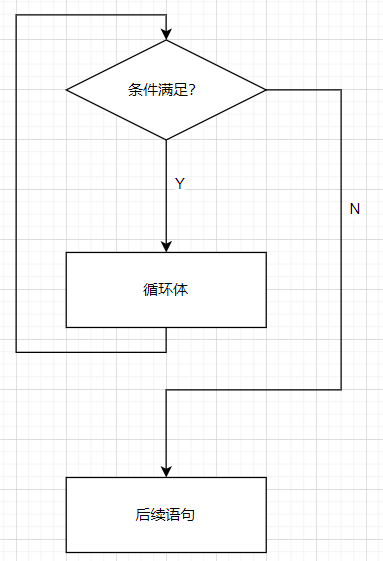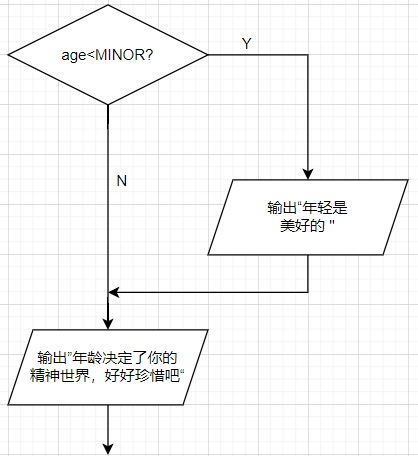while循环：

• 如果我们把while翻译作"当“。那么一个while循环的意思就是：当条件满足时。不断的重复循环体内的语句。
• 循环执行之前判断循环是否继续循环，所有有可能循环一次也没有被执行。
• 条件成立是循环继续的条件。

#include <stdio.h>
int main()
{
int x;
int n=0;
printf("请输入正整数");
scanf("%d",&x);
n++;
x/=10;
while(x>0) {
n++;
x/=10;
}
printf("%d",n);
return 0;
}

1.个位数；

2.10；        特殊的倍数

3.0；          范围两端的数，严格来说0不是正整数。

4.负数。     这里先不验证负数

x       n

10     0

1      1

0      2         循环结束

#include <stdio.h>
int main()
{
int x;
int n=0;
printf("请输入正整数");
scanf("%d",&x);
n++;
x/=10;
while(x>0) {
n++;
x/=10;
printf("x=%d,n=%d\n",x,n);
}
printf("%d",n);
return 0;
}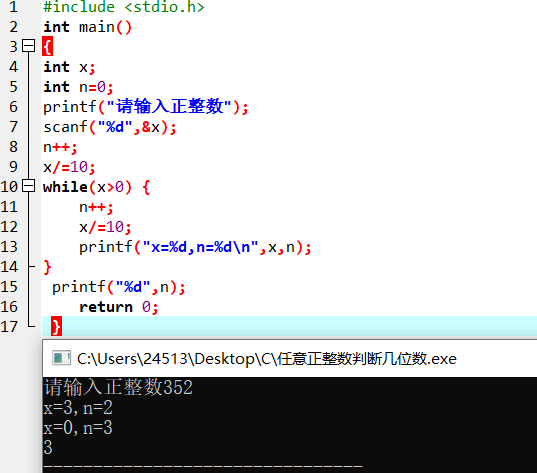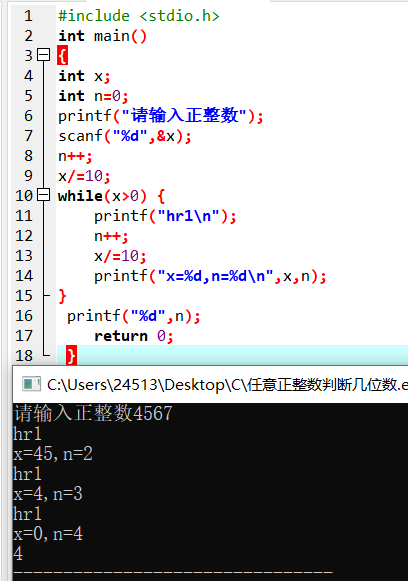1.用户输入x;

2.初始化n为0；

3.x=x/10,去掉个位；

4.n++;

5.如果x>0,回到3；

6.否则n就是结果。

do-while循环

• 再进入循环的时候不做检查，而是在执行完一轮循环体的代码之后，再来检查循环的条件是否满足，如果满足则继续下一轮循环，不满足则结束循环。

do

{

<循环体语句>

}while(<循环条件>);            while后面一定有个分号，表示语句结束。      结束一个语句，要么是大括号要么是分号。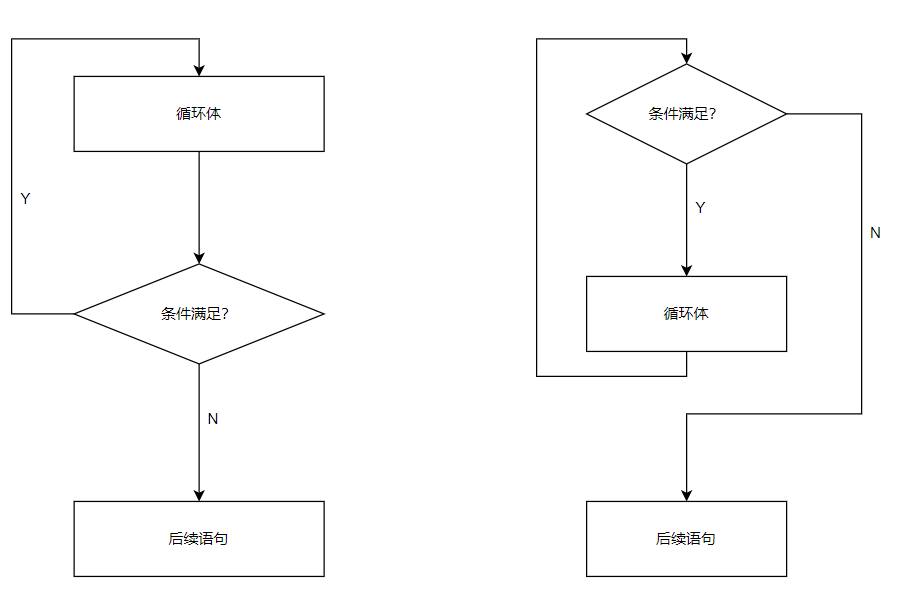do-while循环  (先进循环体）                                                                      while循环（先判断条件）

• do-while循环和while循环很像，区别是在循环体执行结束的时候才来判断条件。也就是说，无论如何，循环都会执行至少一遍，然后再来判断条件。与while循环相同的是，条件满足时执行循环，条件不满足时结束循环。

#include <stdio.h>
int main()
{
int n=0;
int x;
printf("请输入正整数");
scanf("%d",&x);
do{
n++;
x/=10;

while(x>0);

printf("位数：%d",n);
return 0;
}

log2X   即2多少次方等于X

#include <stdio.h>
int main()
{
int x;
int ret=0;
printf("请输入x的值");
scanf("%d",&x);
int t=x;                                                      注：因为X的值一直在变，所以在此处定义了t变量，为了后面 printf("2的%d次方等于%d",ret,t) ;   这是一个小套路：计算之前先保存原始的值，后面可能会用到
while(x>1){
x/=2;
ret++;
}
printf("2的%d次方等于%d",ret,t) ;
return 0;
}

#include <stdio.h>
int main()
{
int count=100;
while(count>=0){

printf("%d\n",count);
count--；
}
printf("发射！\n");
return 0;

}

• 这个循环需要执行多少次？
• 循环停下来的时候，有没有输出最后的0？
• 循环结束后，count的值是多少？

count         printf

3                  3

2                  2

1                  1

0                  0

-1

1.有4个输出，循环走了四轮

2.0有输出

3.当循环结束的时候，count=-1

1.执行101次

2.0有输出

3.循环结束后，count的值是-1

#include <stdio.h>
int main()
{
int count=100;
while(count>=0){

count--；                                      count--； 和 printf("%d\n",count); 交换下位置，再思考下上面三个问题。

printf("%d\n",count);
}
printf("发射！\n");
return 0;

}

count         printf

3

2                  2

1                  1

0                  0

-1                 -1

• 让计算机来想一个数，然后让用户来猜，用户每输入一个数，就告诉他是大了还是小了，直到用户猜中为止，最后还要告诉用户猜了多少次。
• 因为需要不断重复让用户猜，所以需要用到循环。
• 在实际写出程序之前，我们可以先用文字描述程序的思路。
• 核心重点是循环的条件
• 人们往往会考虑循环终止的条件
1. 计算机先想一个数，记在变量number里
2. 一个负责记次数的变量count初始化为0
3. 让用户输入一个数字a
4. count递增(加1）
5. 判断a和number的关系，如果大了，就输出大，如果a小，就输出小了
6. 如果a和number是不相等的，程序返回到第三步                                                      循环的条件是a和number不想等
7. 否则程序输出猜中和次数，然后结束

• 每次召唤rand()就得到一个随机的整数

%100

• x%n的结果是[0,n-1]的一个整数；
• 如：123%100结果是23；

#include <stdio.h>
#include <stdlib.h>
#include <time.h>
int main()
{
srand(time(0));
int number=rand()%100+1;
int count=0;
int a;
printf("我已经想好了一个1到100之间的数呢\n");
do{
printf("再猜猜这个数\n");
scanf("%d",&a);
count++;
if(a>number){
printf("大了!\n");
}else{
printf("小了!\n");
}
}while(a!=number) ;
printf("您用了%d次猜中猜到了这个数\n",count);
return 0;

}

• 让用户输入一系列的正整数，最后输入-1表示输入结束，然后程序计算出这些数字的平均数，输出输入数字的个数和平均值
• 变量-算法-流程图-程序

1. 一个记录读到的整数的变量
2. 平均要怎么算？
• 只需要每读到一个数，就把它加到一个累加的变量里，到全部数据读完，在拿它去除读到数的个数就可以
• 一个变量记录累加的结果，一个变量记录读到数的个数

1. 初始化变量sum和count为0；
2. 读入number;
3. 如果number不是-1，则将number加入sum,并将count+1回到2；
4. 如果number是-1，则计算和打印sum和count(注意换成浮点来计算）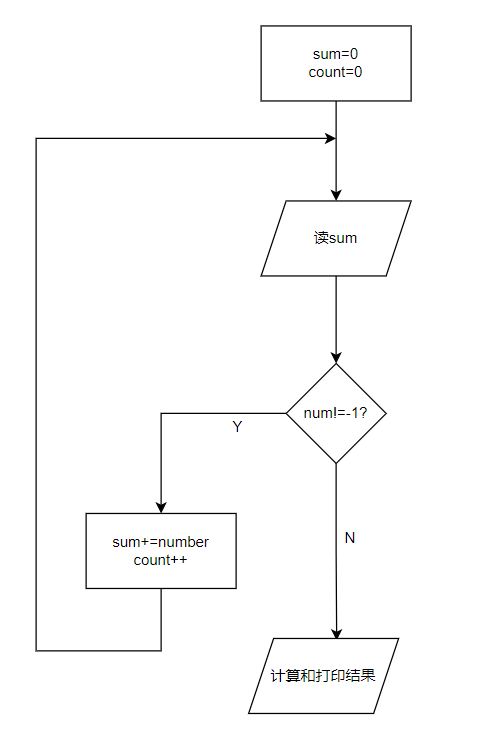do-while循环

#include <stdio.h>
int main()
{
int sum,count=0;
int number;
do{
printf("请输入一个正整数\n");
scanf("%d",&number);
if(number!=-1){
count++;
sum+=number;
}
}while(number!=-1);

printf("平均值为%lf",1.0*sum/count);
return 0;
}

while循环

#include <stdio.h>
int main()
{
int sum,count=0;
int number;
printf("请输入一个正整数\n");
scanf("%d",&number);
while(number!=-1){
count++;
sum+=number;
printf("请输入一个正整数\n");
scanf("%d",&number);                                                没有这句scanf("%d",&number); 程序会死循环
}
printf("平均值为%lf",1.0*sum/count);
return 0;
}

• 一个整数是由1至多位数字组成的，如何分解出整数的各个位上的数字，然后加一计算
• 对一个整数做%10的操作，就得到了它的个位数
• 对一个整数做/10的操作，就去掉了它的个位数
• 然后再做%10，就得到原来数的十位数呢
• 以此类推；
1. 输入一个正整数，输出逆序的数
2. 结尾0的处理

700逆序为007程序：

#include <stdio.h>
int main()
{
int x;
printf("请输入x的值\n");
scanf("%d",&x);
int digit;
int ret;
while(x>0){
digit=x%10;
printf("%d",digit);                注：这里%d后面一定要没有\n，才能输出007
ret=ret*10+digit;                 注：原来的结果往左移一位，加上新的digit
x/=10;
}
//    printf("%d",ret);               使用快捷键ctrl+/快速将该行变为注释
return 0;
}

700逆序为7的程序：

#include <stdio.h>
int main()
{
int x;
printf("请输入x的值\n");
scanf("%d",&x);
int digit;
int ret;
while(x>0){
digit=x%10;
//    printf("%d",digit);
ret=ret*10+digit;
x/=10;
}
printf("%d",ret);
return 0;
}

• n!=1x2x3x4x...xn
• 写一个程序，让用户输入n，然后计算输出n!
• 变量：
• 显然读用户的输入需要一个int 的n,然后计算的结果需要用一个变量保存，可以是int的factor,在计算中需要有一个变量不断的从1递增到n,那可以是int的i

#include <stdio.h>
int main()
{
int n;
printf("请输入n\n");
scanf("%d",&n);
int fact=1;
int i=1;
while(i<=n){
fact=fact*i;
i++;
}
printf("%d!的值为%d",n,fact);
return 0;
}

for循环实现：

#include <stdio.h>
int main()
{
int n;
printf("请输入n\n");
scanf("%d",&n);
int fact=1;                    小套路：做求和的程序时，记录结果的变量应该初始化为0，做乘积的程序时，记录结果的变量应该初始化为1
int i=1;
for(i=1;i<=n;i++){                      i=1是初始条件；i<=n是循环继续的条件；i++是循环每一轮要做的事情
fact*=i;
}
printf("%d!的值为%d",n,fact);
return 0;
}

for循环

• for循环像一个计数循环：设定一个计数器，初始化它，然后在计数器到达某个数之前，重复执行循环体，而每执行一轮循环，计数器值以一定步进进行调整，比如加1或者减1
• for(i=0;i<5;i=i+1){                          注：for循环里三个条件之间用的;

printf("%d",i);

}

for读成对于

• for(count=10;count>0;count--)
• 就读成：对于一开始的count=10,当count大于0时，重复做循环体，，第一轮循环在做完循环体内语句后，使得count--

• for(i=0,i<n,i++)
• 则循环的次数是n，而循环结束以后，i的值是n。循环的控制变量i，是选择从0开始还是从1开始，是判断i<n还是i<=n，对循环的次数，循环结束后变量的值都有影响。

for==while

for(i=1,i<=1,i++){

fact*=1;

}

==

int i=1;

while(i<=n){

fact*=1;

i++;

}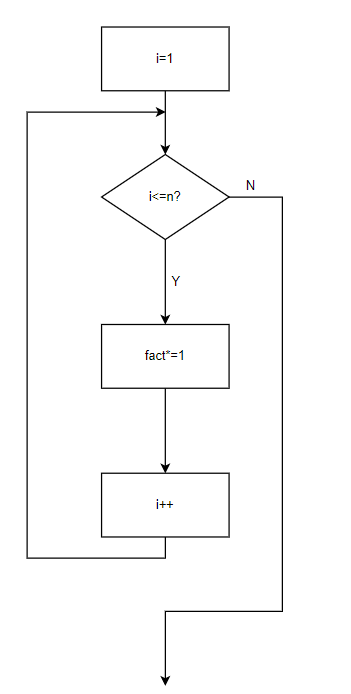for循环

for（初始动作；条件；每轮动作）{

}

• for中的每一个表达式都是可以省略的

Tips:

• 如果有固定次数，用for
• 如果必须执行一次，用do-while
• 其他情况用while

#include <stdio.h>
int main()
{
int x;
printf("请输入X");
scanf("%d",&x);
int i;
int isPrime=1;
for(i=2;i<x;i++){
if(x%i==0){
isPrime=0;
break;                                   跳出循环
}
}
if(isPrime==0){
printf("不是素数");
}else{
printf("是素数");
}
return 0;
}

break&continue

• break:跳出循环
• continue：跳出循环这一轮剩下的语句，进入下一轮

#include <stdio.h>
int main()
{
int x;
int i;

for(x=1;x<100;x++) {
int isPrime=1;

for(i=2;i<x;i++){
if(x%i==0){
isPrime=0;
break;
}
}
if(isPrime==1){
printf("%d\n",x);
}
}
return 0;
}

#include <stdio.h>
int main()
{
int x;
int i;
int cnt=0;

for(x=1;cnt<50;x++) {
int isPrime=1;

for(i=2;i<x;i++){
if(x%i==0){
isPrime=0;
break;
}
}
if(isPrime==1){
cnt++;
printf("%d\t",x);
if(cnt%5==0){                  注：每5个数字一行
printf("\n");
}
}
}
return 0;
}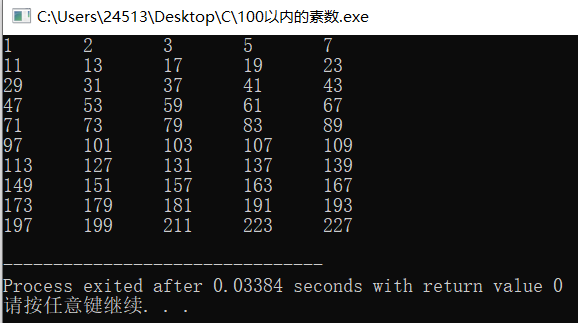#include <stdio.h>
int main()
{
int x=1;
int a;
int b;
int c;
for(a=1;a<x*10;a++){
for(b=1;b<x*10/2;b++){
for(c=1;c<c*10/5;c++){
if(a+2*b+5*c==x*10) {
printf("%d个一角加%d个2角加%d个5角等于%d块钱\n",a,b,c,x);
}
}
}
}
return 0;
}

int main()
{
int x=5;
int a;
int b;
int c;
int exit=0;
for(a=1;a<x*10;a++){
for(b=1;b<x*10/2;b++){
for(c=1;c<c*10/5;c++){
if(a+2*b+5*c==x*10) {
printf("%d个一角加%d个2角加%d个5角等于%d块钱\n",a,b,c,x);
exit=1;
break;                   离开第一个for循环

}
}
if(exit==1){
break;              离开第二个for循环

}
}
if(exit==1){
break;               离开第三个for循环

}
}
return 0;
}

break和continue  只能对它所在的那层循环做

goto

#include <stdio.h>
int main()
{
int x=5;
int a;
int b;
int c;

for(a=1;a<x*10;a++){
for(b=1;b<x*10/2;b++){
for(c=1;c<c*10/5;c++){
if(a+2*b+5*c==x*10) {
printf("%d个一角加%d个2角加%d个5角等于%d块钱\n",a,b,c,x);
goto out;                           跳到out这个位置
}
}

}

}
out:                                  out位置在这里
return 0;
}

1.求和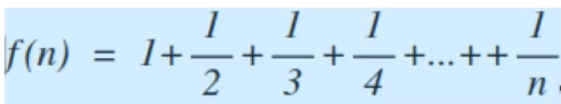#include <stdio.h>
int main()
{
int n=100;
int i;
double sum=0;
for(i=1;i<=n;i++){
sum+=1.0/i;                      在这里，如果是1/i，则值一定是0。所以写成1.0/i，两个数相除，如果其中一个是浮点数，那个另一个也会变成浮点数，结果也是浮点数。
}
printf("f(%d)=%f",n,sum);
return 0;
}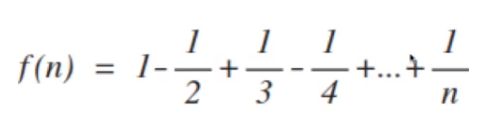#include <stdio.h>
int main()
{
int n=10;
int i;
double sum=0;
double sign=1;
for(i=1;i<=n;i++){
sum+=sign/i;
sign=-sign;
}
printf("f(%d)=%f",n,sum);
return 0;
}

#include <stdio.h>
int main()
{
int x=3234;
int i;
do{
i=x%10;
printf("%d ",i);                  %d后面有个空格
x/=10;
}while(x>0);
return 0;
}

#include <stdio.h>
int main()
{
int x=3234;
int i;
do{
i=x%10;
printf("%d",i);
if(x>9){
printf(" ");
}

x/=10;
}while(x>0);
printf("\n");
return 0;
}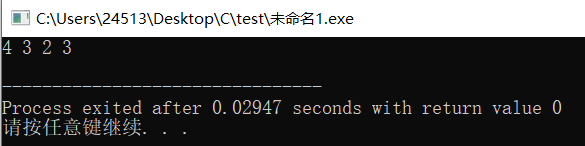#include <stdio.h>
int main()
{
int x=3234;
int i;
int t=0;
int y;
do{
y=x%10;
t=t*10+y;
x/=10;
}while(x>0);
printf("t=%d\n",t);                           逆序

x=t;
do{
i=x%10;
printf("%d",i);
if(x>9){
printf(" ");
}
x/=10;
}while(x>0);
printf("\n");
return 0;
}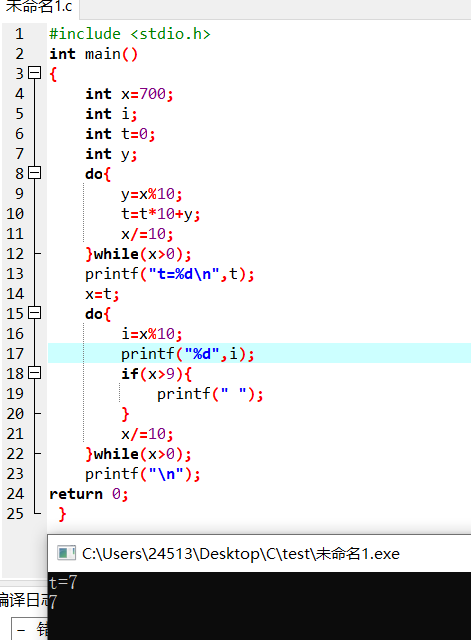700输出为7 0 0

#include <stdio.h>
int main()
{
int x=700;
int t;
int a=x;
int cnt=0;
do{
a/=10;
cnt++;
}while(a>0);
do{
printf("%d",t);
printf(" ");
}
return 0;
}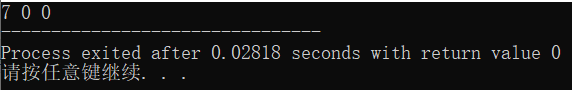#include <stdio.h>
int main()
{
int x=700;
int t;
int a=x;
int cnt=0;
do{
a/=10;
}while(a>9);
do{
printf("%d",t);
printf(" ");
}
return 0;
}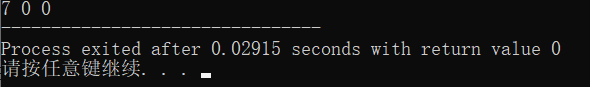do-while循环改为while循环

#include <stdio.h>
int main()
{
int x=1;
int t;
int a=x;
int cnt=0;
while(a>9){
a/=10;
}
do{
printf("%d",t);
printf(" ");
}
return 0;
}

#include <stdio.h>
int main()
{
int a;
int b;
int min;
int i=1;
int ret=0;
scanf("%d %d",&a,&b);
if(a<b){
min=a;
}else{
min=b;
}
for(i=1;i<min;i++)
{
if(a%i==0){
if(b%i==0){
ret=i;
}
}
}
printf("最大公约数为%d",ret);
return 0;
}

1.如果b等于0，计算结束，a就是最大公约数；

2.否则计算a/b的余数，让a等于b，而b等于那个余数；

3.回到第一步。

#include <stdio.h>
int main()
{
int a,b;
int t;
scanf("%d %d",&a,&b);
while(b!=0){
t=a%b;
a=b;
b=t;

}
printf("gcd=%d",a);
return 0;
}

TAG